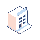• Fiyatlar

# Odoo 13 - sequence/priority/order of executing compute functions in the same model

Hi everyone,

I wonder if there's a way to set the order of executing the compute functions in the same model?

model1:

- field1

- field2

- compute_field1 (stored, compute_func1, depends on field1)

- compute_field2 (stored, compute_func2, depends on field2)

- compute_field3 (stored, compute_func3, depends on compute_field1, compute_field2)

For some reason, when field1 and field2 are changes, Odoo launches compute_func1, then compute_func3 and then compute_func2, but doesn't fire compute_func3 again, hence compute_field3 doesn't get the final correct value.

So, how do I tell Odoo the order/priority/sequence of launching compute functions?

PS: I know that the simplest solution would be to combine those functions into one, and I've done that already, however in my case, the setup with 3 functions above would be more optimized in the mean of speed and performance.